# Creating Data Porn: A brief tutorial¶

There are lots of options for data visualization. They generally trade-off the ease of use and the configurability needed to produce polished graphs for publication. In my daily workflow, I use different options for different purposes. During analysis, I prefer quick and dirty approaches that allow me to see the data, but I approaches that give me better control when I want to create figures for a paper. This also sets some expectation. While a simple visualisation during the analysis should only take one or two lines of code, the finished figures usually require quite a bit of code to make them look just right. For this tutorial, I will focus on making publication-ready figures.

I tried different languages and different packages for creating figures. In my experience, the native R plot and the commonly used ggplot package do not provide sufficient control. I never found a way to define the exact dimensions and resolution of a figures, which is often necessary for creating figures according to journal specification. Instead, I use the matplotlib and seaborn packages for Python. There syntax is relatively easy and they provide full control over the aestethics.

## Getting started¶

If you never used Python before, it might seem a bit daunting. But using modern Python distributions, makes it really easy to get started.

Download anaconda: Anaconda is a Python distribution that comes with installers for all major operating systems. You can download it from here: https://www.anaconda.com/distribution/

Install additional packages: There are many additional packages that extend the functionality of Python. Anaconda comes with a set of the most commonly used packages for science, but I recommend one additional one for plotting, namely seaborn. To install it, open a terminal window and type "conda install seaborn"

## Example: Clustering¶

In this tutorial, we will go through some steps to visualize clustering solutions. I'll use the iris dataset, because it is a commonly used and relatively easy to use dataset but the same methods should apply to other datasets, e.g. using behavioural or cognitive data.

Show the plots within the notebook:

In :
%pylab inline

Populating the interactive namespace from numpy and matplotlib


We are loading the iris data using the convenience function in sklearn.

In :
import pandas as pd

In :
from sklearn.datasets import load_iris
features = pd.DataFrame(data.data)
cluster_labels = data.target


## Plotting profiles¶

First, we may want to show the feature profile of each cluster

In :
import matplotlib.pyplot as plt
import numpy as np
import pandas as pd
from scipy.stats import sem, zscore
import seaborn as sns


A few definitions help to define the plot and make it publication-ready

In :
sns.set_style('white')
from matplotlib import rcParams
rcParams['font.family'] = 'serif'
rcParams['font.serif'] = ['CMU Serif']   # define the font for the plots
rcParams['text.usetex'] = True  # using Latex as a backend creates better type setting
rcParams['axes.labelsize'] = 9  # define the font size
rcParams['xtick.labelsize'] = 9
rcParams['ytick.labelsize'] = 9
rcParams['legend.fontsize'] = 9
mm2inches = 0.039371


Shaping the data for plotting

In :
features = features.apply(zscore)

In :
features.head()

Out:
0 1 2 3
0 -0.900681 1.019004 -1.340227 -1.315444
1 -1.143017 -0.131979 -1.340227 -1.315444
2 -1.385353 0.328414 -1.397064 -1.315444
3 -1.506521 0.098217 -1.283389 -1.315444
4 -1.021849 1.249201 -1.340227 -1.315444
In :
means = features.groupby(cluster_labels).apply(np.mean)
SEs = features.groupby(cluster_labels).apply(sem)

In :
plt.figure(figsize=[75*mm2inches, 100*mm2inches], dpi=300)
colours = ["#3B4992FF", "#EE0000FF", "#008B45FF"]

for i, cluster in enumerate(np.unique(cluster_labels)):
plt.errorbar(features.columns, means.iloc[cluster, :].values, yerr=SEs.iloc[cluster],
linewidth=2, capsize=2, elinewidth=1, markeredgewidth=1, color=colours[i])

plt.ylim([-2, 2])
plt.ylabel('z-score')
sns.despine(offset=8)

ax = plt.gca()
ax.set_xticks(np.arange(0, len(features.columns)))
ax.set_xticklabels(data['feature_names'], rotation='90')
ax.set_yticks(np.linspace(-2, 2, 5))

legend = plt.legend(np.unique(cluster_labels), bbox_to_anchor=(1.05, 1.05), frameon=True)
legend.get_frame().set_edgecolor('k')
legend.get_frame().set_linewidth(0.5)

plt.axhline(0, linestyle='solid', linewidth=0.5, color='k')
plt.axhline(1, linestyle='dashed', linewidth=0.5, color='k')
plt.axhline(-1, linestyle='dashed', linewidth=0.5, color='k')

Out:
<matplotlib.lines.Line2D at 0x12194d8d0>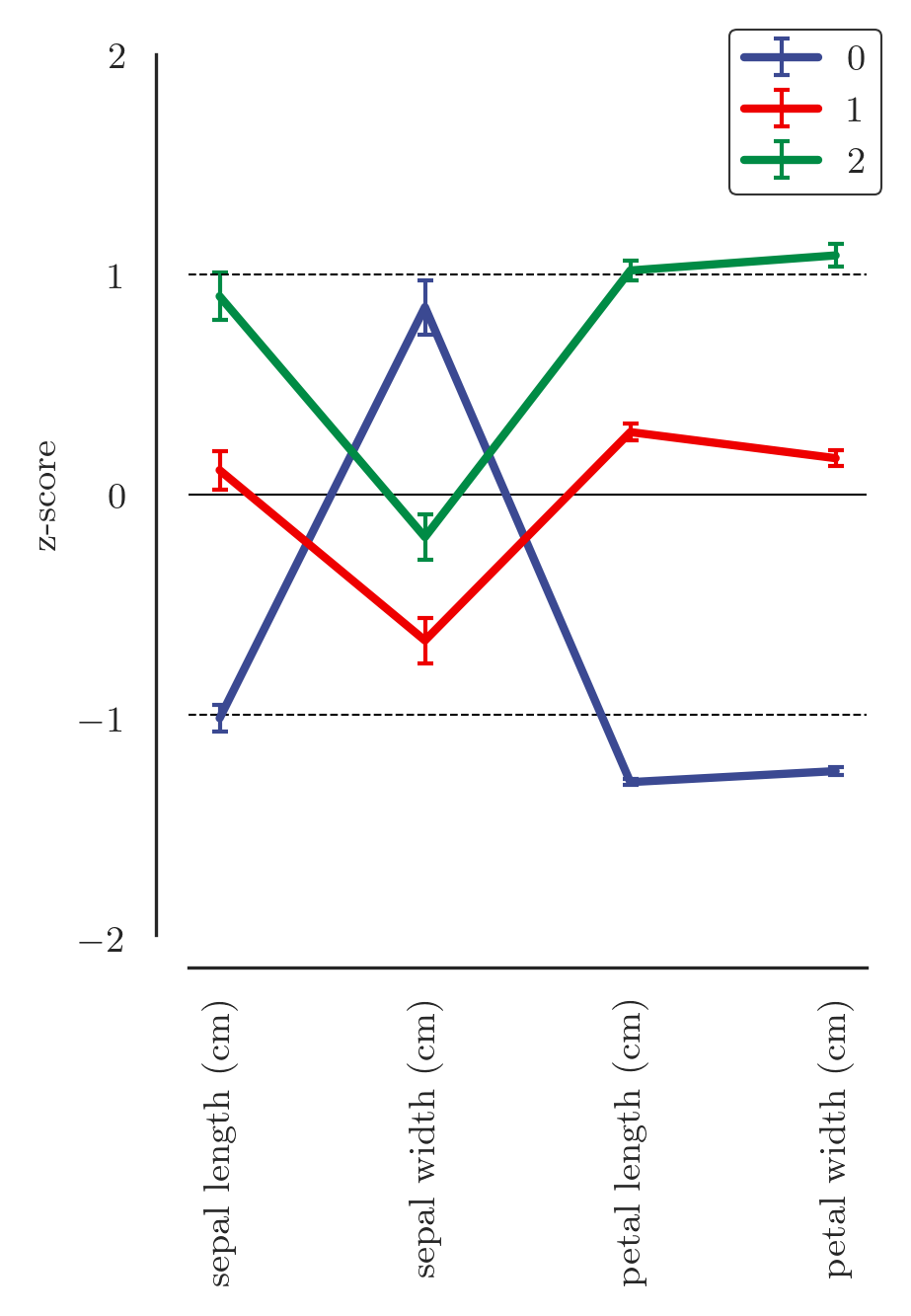Note on colour aesthetics: Creating effective colour maps is not an easy task. You want colours that work well together, create contrasts, and are accessible to colour-blind individuals. For most plots, I use colour maps from this project: https://nanx.me/ggsci/. For plots that require continuous colour scales, I use maps from the viridis project that are integrated in matplotlib. These are designed to be perceptually continuous (read more here: https://www.r-bloggers.com/ggplot2-welcome-viridis/)

### Playing with the code¶

You can play around with the code by commenting out some of the commands and observing the effect. You can either comment out a single line with a hash (#) or multiple lines with """

In :
plt.figure(figsize=[75*mm2inches, 100*mm2inches], dpi=300)
colours = ["#3B4992FF", "#EE0000FF", "#008B45FF"]

for i, cluster in enumerate(np.unique(cluster_labels)):
plt.errorbar(features.columns, means.iloc[cluster, :].values, yerr=SEs.iloc[cluster],
linewidth=2, capsize=2, elinewidth=1, markeredgewidth=1, color=colours[i])

plt.ylim([-2, 2])
#plt.ylabel('z-score')
sns.despine(offset=8)

ax = plt.gca()
ax.set_xticks(np.arange(0, len(features.columns)))
ax.set_xticklabels(data['feature_names'], rotation='90')
ax.set_yticks(np.linspace(-2, 2, 5))

"""
legend = plt.legend(np.unique(cluster_labels), bbox_to_anchor=(1.05, 1.05), frameon=True)
legend.get_frame().set_edgecolor('k')
legend.get_frame().set_linewidth(0.5)
"""

plt.axhline(0, linestyle='solid', linewidth=0.5, color='k')
plt.axhline(1, linestyle='dashed', linewidth=0.5, color='k')
plt.axhline(-1, linestyle='dashed', linewidth=0.5, color='k')

Out:
<matplotlib.lines.Line2D at 0x121de6650>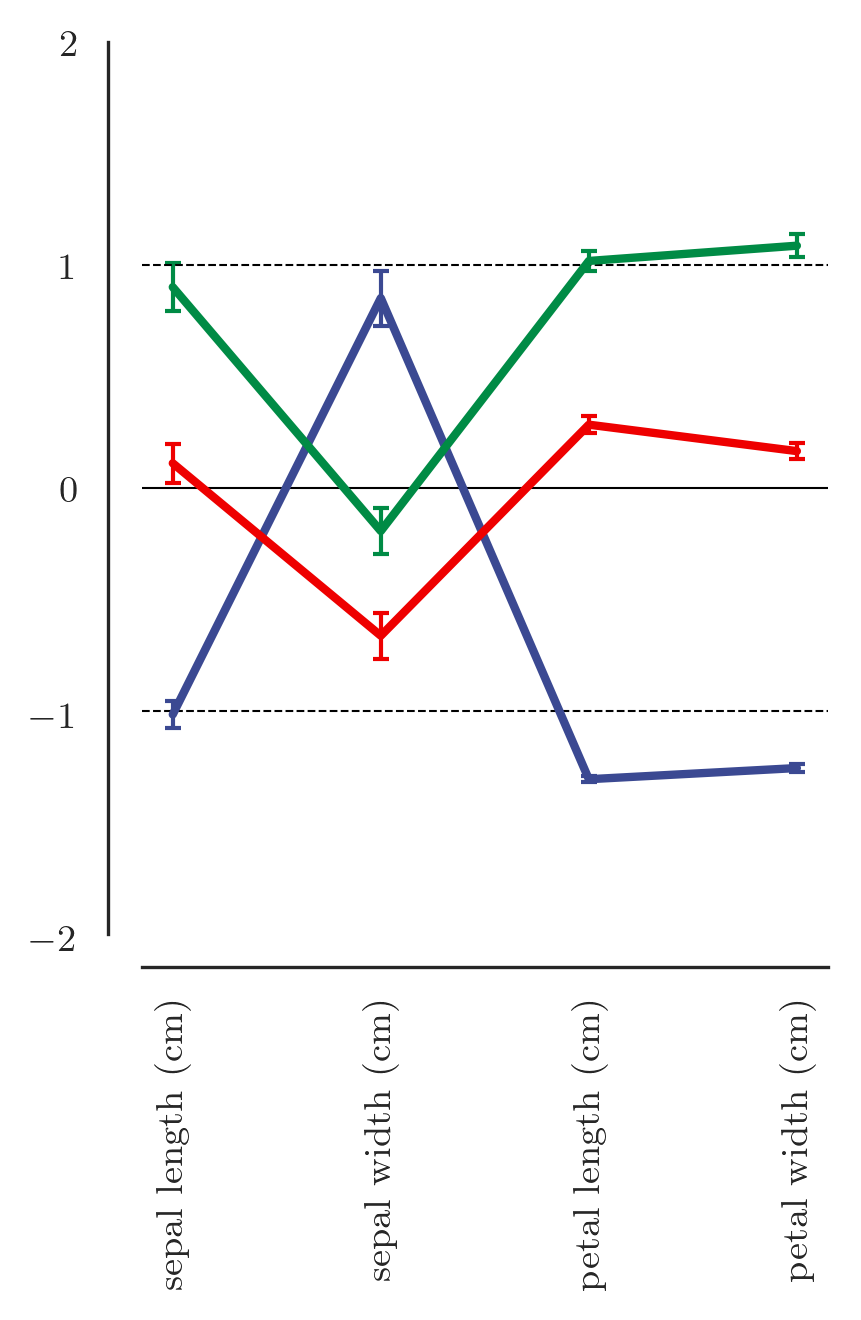## Plotting a distance matrix¶

In :
from sklearn.metrics.pairwise import euclidean_distances
matrix = euclidean_distances(features.values)


Sorting the correlation matrix according to the grouping

In :
sorting_array = sorted(range(len(cluster_labels)), key=lambda k: cluster_labels[k])
sorted_array = matrix
sorted_array = sorted_array[sorting_array, :]
sorted_array = sorted_array[:, sorting_array]

In :
import matplotlib.patches as patches

plt.figure(figsize=[100*mm2inches, 75*mm2inches], dpi=300)

sns.heatmap(sorted_array, vmin=0, cbar=True, cmap='viridis', square=True,
cbar_kws={'label': 'Euclidean distance'})

ax = plt.gca()
ax.set_xticks([]);
ax.set_yticks([]);

# Create a Rectangle patch
for cluster in np.unique(cluster_labels):
x = 0 + np.where(np.asarray(sorted(cluster_labels)) == cluster)
y = 0 + np.where(np.asarray(sorted(cluster_labels)) == cluster)
width = height = np.where(np.asarray(sorted(cluster_labels)) == cluster)[-1] + 1 - np.where(np.asarray(sorted(cluster_labels)) == cluster)
rect = patches.Rectangle((x, y),
width,
height,
linewidth=0.5, edgecolor='w', facecolor='none')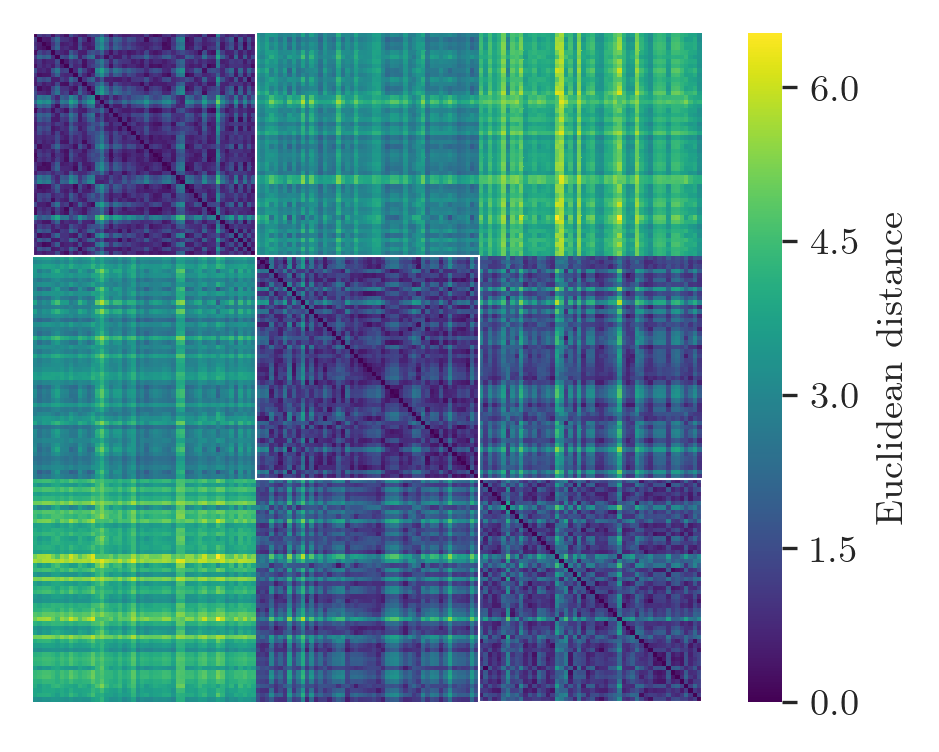This is only a very basic demonstration of the plots that you can generate with matplotlib and seaborn. The best way to get started is to use the examples on the respective websites and adjust the code to your purposes.

Dedicated Python courses:

Books:

## Further visualization: Gephi¶

There are some native packages for network visualization in Python that do a pretty decent job for simple plots, e.g. :

In :
import bct
import networkx as nx

colours = ["#3B4992FF", "#EE0000FF", "#008B45FF"]

matrix = matrix/matrix.max()
matrix = 1-matrix
plotting_matrix = bct.threshold_proportional(matrix, 0.1)

G = nx.from_numpy_matrix(plotting_matrix)

for community in np.unique(cluster_labels):

nx.draw_networkx_nodes(G,pos,
nodelist=np.where(cluster_labels == community).tolist(),
node_color=colours[int(community)-1],
node_size=40,
alpha=0.8)

nx.draw_networkx_edges(G,pos,width=0.1,alpha=0.5)
plt.axis('off');
plt.show();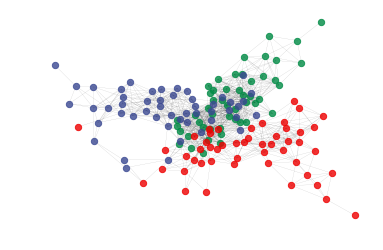However, there are much fancier ways of plotting graphs available from a dedicated application called Gephi. You can download it for free here: https://gephi.org

Gephi expects the data to be in a certain shape. First, we need to reshape the distance matrix:

In :
pd.DataFrame(matrix).head()

Out:
0 1 2 3 4 5 6 7 8 9 ... 140 141 142 143 144 145 146 147 148 149
0 1.000000 0.819856 0.870491 0.830965 0.960158 0.840957 0.898714 0.959218 0.751763 0.852530 ... 0.337859 0.356229 0.436421 0.334120 0.330488 0.361335 0.375858 0.417181 0.414004 0.489217
1 0.819856 1.000000 0.919843 0.933531 0.787653 0.665937 0.847051 0.857495 0.900740 0.958465 ... 0.334264 0.350408 0.489826 0.320312 0.308712 0.367358 0.439431 0.426183 0.384723 0.507839
2 0.870491 0.919843 1.000000 0.956521 0.848136 0.716066 0.923852 0.908488 0.880159 0.942294 ... 0.310204 0.324293 0.452621 0.298965 0.292398 0.338777 0.391507 0.397098 0.376242 0.482284
3 0.830965 0.933531 0.956521 1.000000 0.808531 0.678255 0.891977 0.870784 0.919843 0.940790 ... 0.310098 0.322047 0.466012 0.297247 0.289004 0.339630 0.404553 0.399179 0.372433 0.488438
4 0.960158 0.787653 0.848136 0.808531 1.000000 0.862143 0.895652 0.928953 0.729254 0.821392 ... 0.321043 0.337905 0.413555 0.318714 0.317486 0.342026 0.348644 0.397225 0.404147 0.470447

5 rows × 150 columns

In :
pd.DataFrame(matrix).to_csv('/Users/joebathelt1/Desktop/test_gephi.csv')


Notice that the rows and columns have the same name. That is required for Gephi.

Next, we produce a second file with information about the cluster membership:

In :
pd.DataFrame(vstack([pd.DataFrame(matrix).index, cluster_labels]).transpose(), columns=['ID', 'group']).head()

Out:
ID group
0 0 0
1 1 0
2 2 0
3 3 0
4 4 0
In :
pd.DataFrame(vstack([pd.DataFrame(matrix).index, cluster_labels]).transpose(), columns=['ID', 'group']).to_csv('/Users/joebathelt1/Desktop/test_gephi_groups.csv', index=False)


This table contains one column with the ID (same as in the distance matrix) and a column with the associated cluster of that node.

In :
from IPython.display import Image


Import the distance matrix

In :
Image('./Gephi_steps/Step1.png')

Out: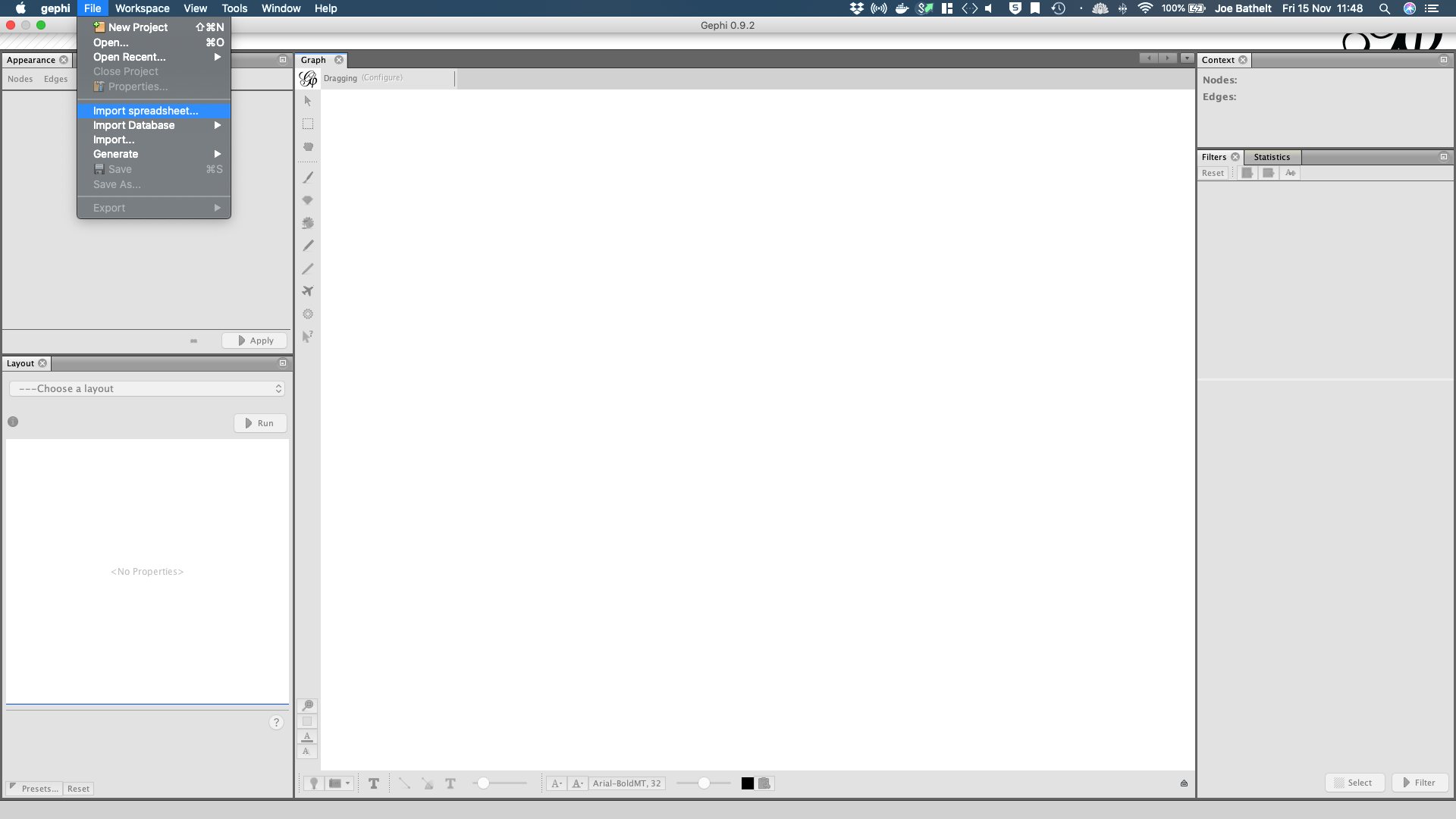In :
Image('./Gephi_steps/Step2.png')

Out: